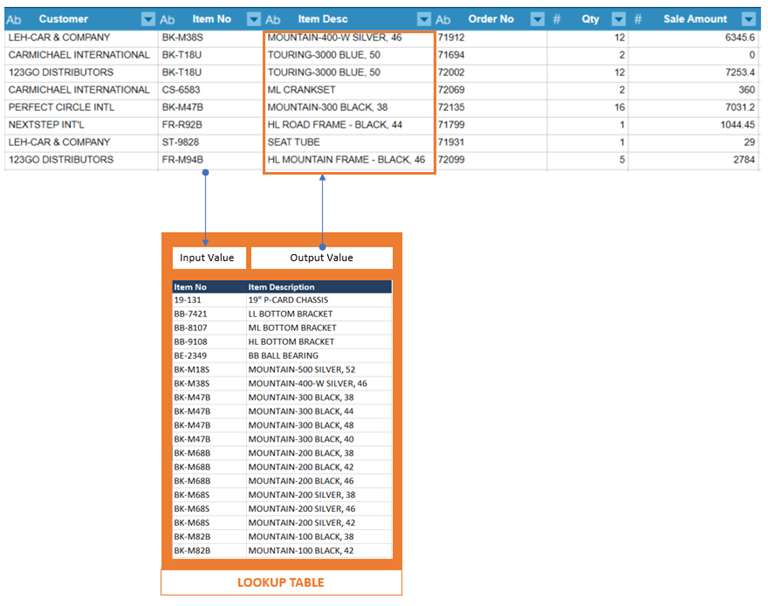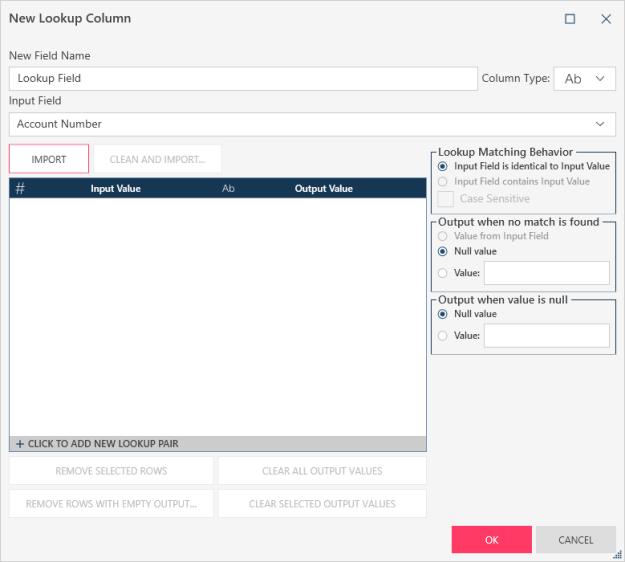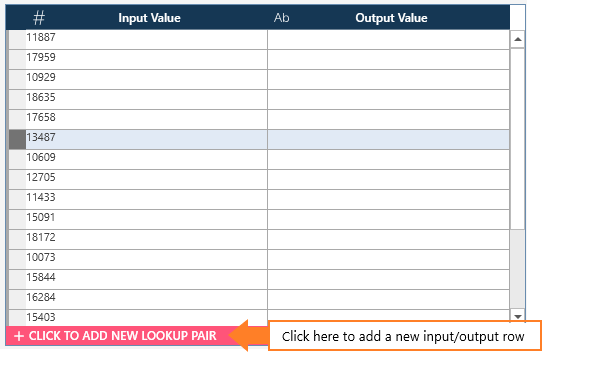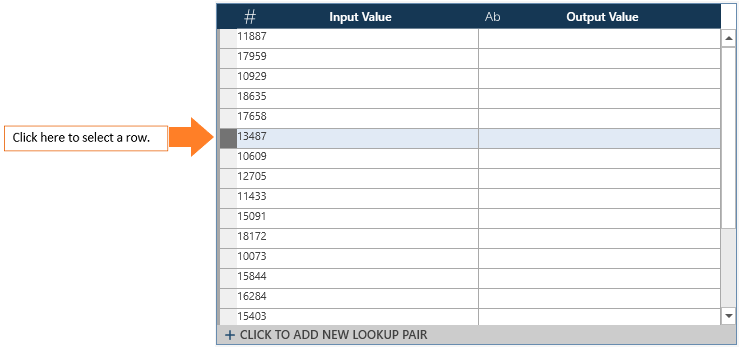Altair® Monarch®

# Creating New Lookup Columns

A lookup calculated field provides a convenient table lookup where the value of a designated input field is checked against a lookup table. If a matching input value from the lookup table is found, then the corresponding output value is used. If no matching value is found, then the output is either the same as the input value, null or a default value. Comparisons can be case-insensitive or case-sensitive.

For example, you can have a report that just contains an Item No field. You can define a lookup field, Item Desc, that will match an Item No value with the corresponding Item Description:The central control for the lookup field is the lookup table. This is a table where you define the input value/output value pairs. In our example above, the Item Description that matches an Item number.

The table may be populated in two ways:

• You can type in or import the input values

• You can type in or paste the output values.

#### Steps:

1. Right-click on a column and select Column > New Lookup Column.

2. The Create Lookup Field dialog box displays:

3.4. Enter a field name and set the data type.

5. Define the Input and Output Value pairs.

6. If you want to import the Input Field values from a column in the table, select the column from the Input Field drop-down, and then select one of the following buttons:

• Import imports values as is.

• Clean and import cleans up the values before importing. You can select to change the case and/or trim spaces of the input field values.

For each input value, define the corresponding output value.

If you do not want to import input values, or if the input values are incomplete, enter the lookup pairs manually. To do so, select + Click to Add a New Lookup Pair at the bottom of the lookup table.You can fill out the lookup table via a combination of importing and manually enter values.

Use the  following buttons below the lookup table to clear input or output values:

• Remove selected rows. You must select rows before you can select this option. Select the empty space before a row to select it:Use CTRL + Select to select multiple rows.

• Remove rows with empty values

• Clear all output values

• Clear selected output values. You must select output values before you can select this option. Use CTRL + Select to select multiple output values.

7. Define the lookup matching behavior. Select one of the following:

• The input field value must be identical to the input value.

• The input field value must exactly match the input value.

Check the Case Sensitive box to indicate that the input values must match the case of the input field.

8. Define the behavior when no matched is found. Select one of the following:

• Use the input field value when no match is found.

• Output a null value (i.e., "null") when no match is found.

• Use a default text value. If you select this option, enter the text value in the Value box.

9. Define the output when the input value is null. Select one of the following:

• Output a null value (i.e., "null") when no match is found.

• Use a default text value. If you select this option, enter the text value in the Value box.

10. Click OK.